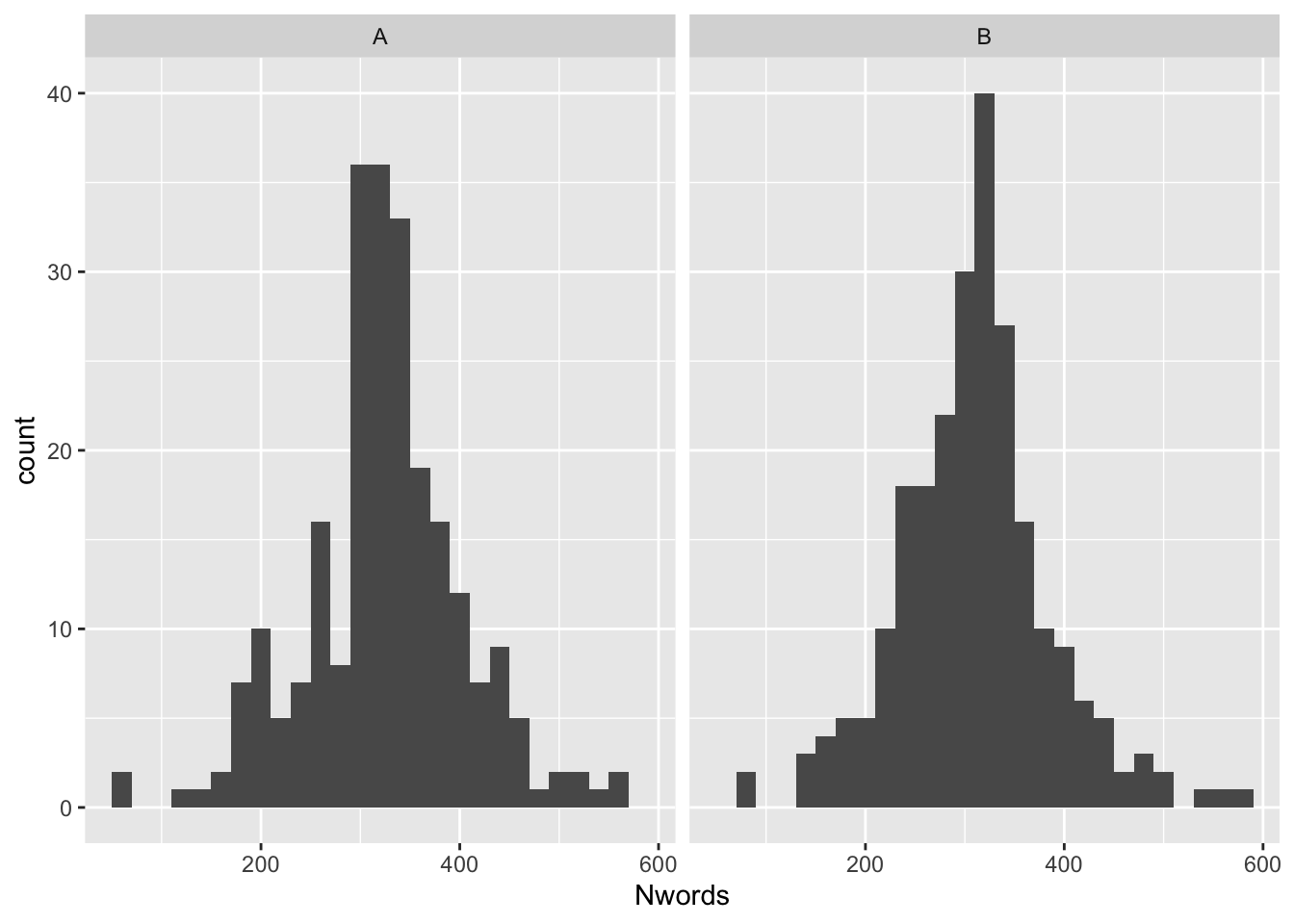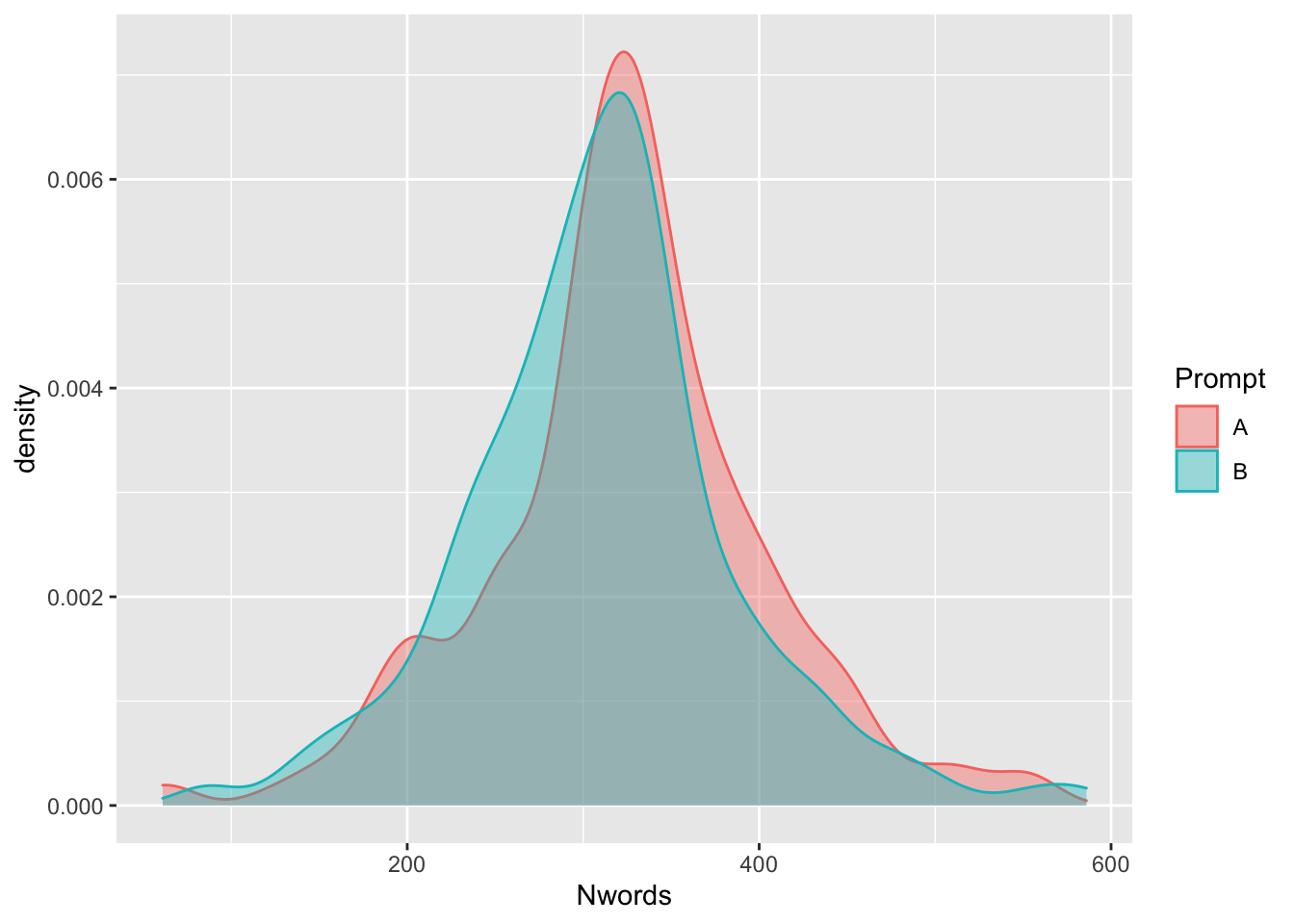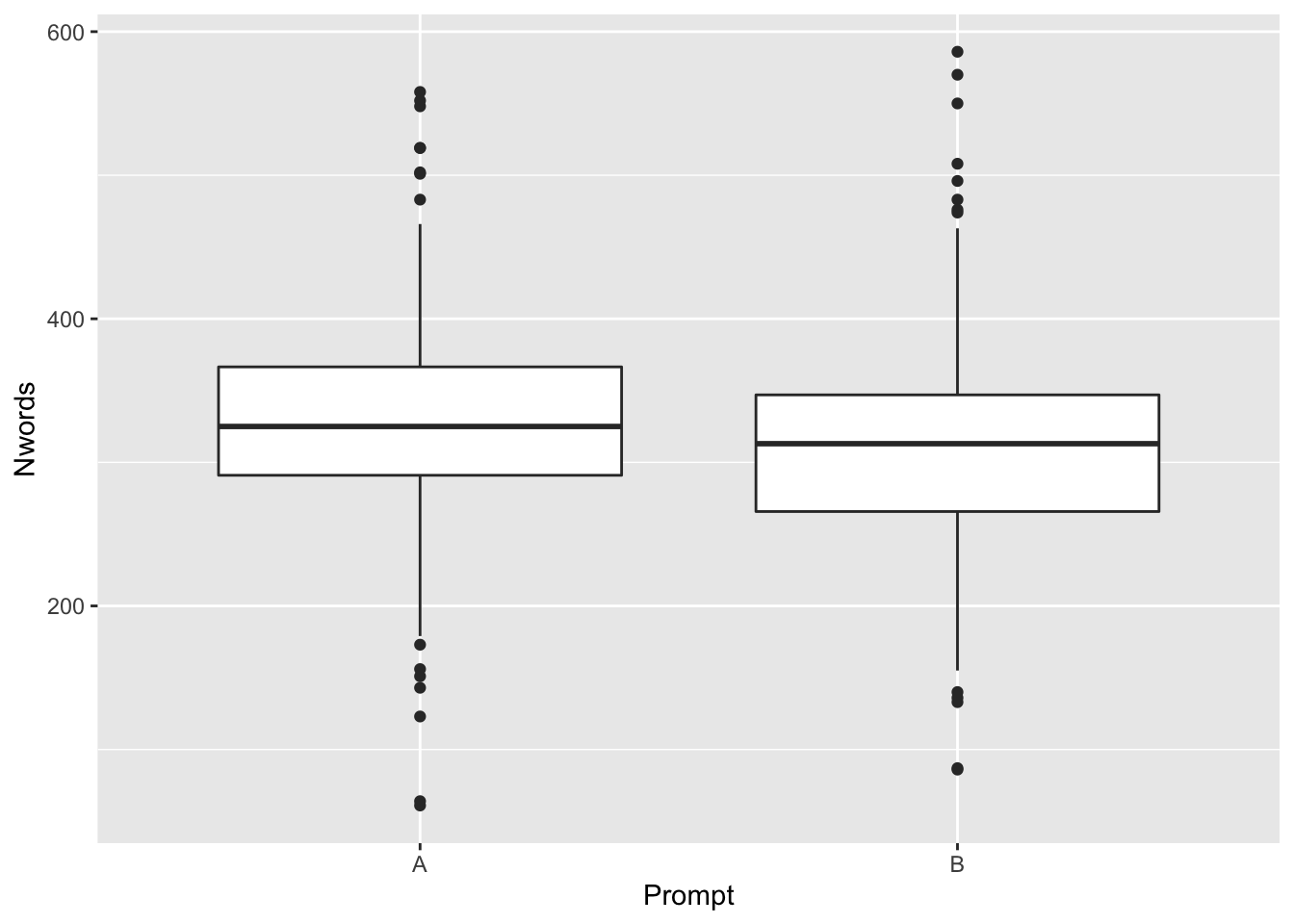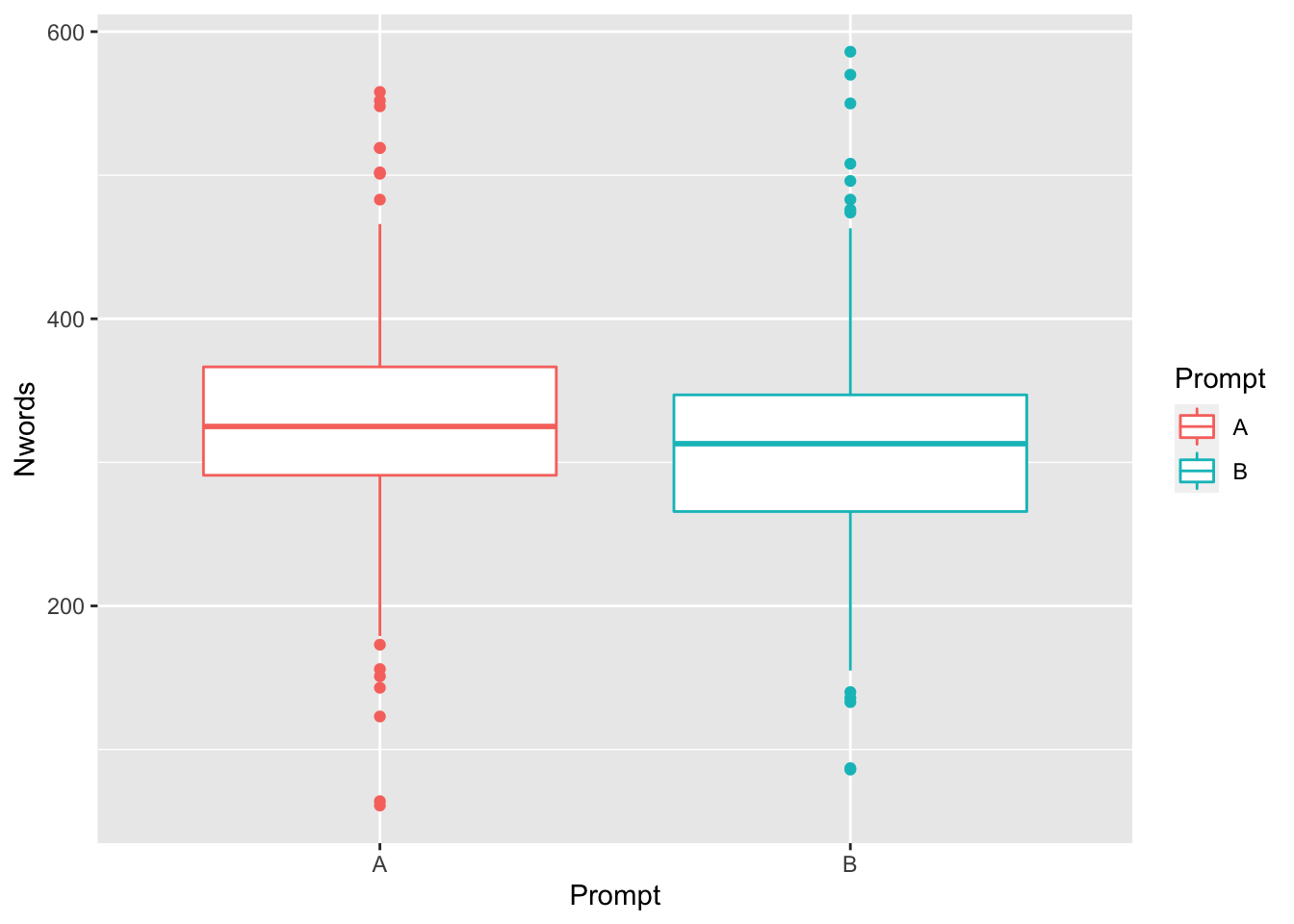## Measuring differences between two independent samples (t-test, Wilcoxon test)

In applied linguistics research, we sometimes want to know whether two independent groups (e.g., intact classes) differ with regard to some measure (motivation, vocabulary knowledge, writing skill, etc.). We also often want to know whether one teaching method works better than another method with regard to some outcome (e.g., vocabulary test score, writing quality score, etc.). In order to determine whether two groups differ in some regard (i.e., to address the first issue outlined above), we can use an independent samples t-test (for parametric data) or a Wilcoxon test (for non-parametric data). In order to determine whether one teaching method works better than another we will need a different set of statistical tests (stayed tuned!), but we could use an indepedent samples t-test to determine whether two groups were similar with regard to some variable prior to testing a teaching method.

In this tutorial, we will be looking at argumentative essays written in response to two prompts and determining whether the essays differ with regard to number of words. In short, we will be addressing the following research question:

Do the responses to the two essay prompts (prompt A and prompt B) differ with regard to number of words?

Our null hypothesis will be that there is no difference in number of words between the two prompts.

### Conducting an independent samples t-test: Assumptions

Independent samples t-tests are rather simple tests that use the sample means and the variance in each sample to determine the probability that the two samples are part of the same population.

Following are the assumptions for an independent samples t-test:

• Each sample is normally distributed
• The variance is roughly equal across samples
• The data do not represent repeated measures (e.g., pre- and post- test scores from the same individuals)
• The data is continuous
• There is only one comparison (an ANOVA is appropriate for multiple comparisons, stay tuned)

### Checking assumptions

Let’s load some data (this is the dataset that we used in class on Wednesday) and check assumptions.

``````mydata <- read.csv("data/distribution_sample.csv", header = TRUE)
summary(mydata)``````
``````##  Prompt      Score           Nwords        Frequency
##  A:240   Min.   :1.000   Min.   : 61.0   Min.   :2.963
##  B:240   1st Qu.:3.000   1st Qu.:273.0   1st Qu.:3.187
##          Median :3.500   Median :321.0   Median :3.237
##          Mean   :3.427   Mean   :317.7   Mean   :3.234
##          3rd Qu.:4.000   3rd Qu.:355.2   3rd Qu.:3.284
##          Max.   :5.000   Max.   :586.0   Max.   :3.489``````

#### Step 1: Check for normality:

First, we will visually inspect the data using histograms.

``library(ggplot2)``
``## Warning: package 'ggplot2' was built under R version 3.6.2``
``````ggplot(mydata, aes(x=Nwords)) +
geom_histogram(binwidth = 20) +
facet_wrap(~Prompt)``````Alternatively, we could also use density plots, which show similar information as histograms, but add smoothing lines. Again, the plot indicates that both datasets are roughly (but not perfectly) normal.

``````ggplot(mydata, aes(x=Nwords, color = Prompt, fill=Prompt)) +
geom_density(alpha = 0.4)``````We can also use the (rather stringent) Shapiro-Wilk test. As we see below, the Shapiro-Wilk test indicates that the data from both prompts significantly vary from a normal distribution.

``````#load dplyr package, which helps us manipulate datasets:
library(dplyr)``````
``## Warning: package 'dplyr' was built under R version 3.6.2``
``````##
## Attaching package: 'dplyr'``````
``````## The following objects are masked from 'package:stats':
##
##     filter, lag``````
``````## The following objects are masked from 'package:base':
##
##     intersect, setdiff, setequal, union``````
``````#create a new dataframe that includes only responses to Prompt A:
promptA <- mydata %>% filter(Prompt == "A")
##create a new dataframe that includes only responses to Prompt b:
promptB <- mydata %>% filter(Prompt == "B")

#Test normality for Nwords in PromptA
shapiro.test(promptA\$Nwords) #p = 0.001872``````
``````##
##  Shapiro-Wilk normality test
##
## data:  promptA\$Nwords
## W = 0.98008, p-value = 0.001872``````
``````#Test normality for Nwords in PromptB
shapiro.test(promptB\$Nwords) #p = 0.0005323``````
``````##
##  Shapiro-Wilk normality test
##
## data:  promptB\$Nwords
## W = 0.9766, p-value = 0.0005323``````

#### Step 2: Checking the assumption of equal variance

Much like the assumption of normalilty, we can check the assumption of equal variance (usually referred to as “homogeneity of variance”) both visually and with a statistical test (e.g., Levene’s test).

We can get an idea of the variance in distribution plots, but one of the the clearest ways to examine the variance is using a boxplot. Below, we see that the variance appears to be similar across the two prompts. (Note, the boxes represent the middle 50% of the data, the line within each box represents the median value. The boxes are roughly the same size, which indicates that the variance is roughly equal).

``````ggplot(data = mydata) +
geom_boxplot(mapping = aes(x = Prompt, y = Nwords))``````In addition to visualizing our data, we can run Levene’s test, which is available via the car() package. The results below indicate that the two variance in Nwords across the two Prompts in our dataset are not significantly different (p = 0.769). In other words, we very clearly meet the assumption of equal variance.

``library(car)``
``## Loading required package: carData``
``````##
## Attaching package: 'car'``````
``````## The following object is masked from 'package:dplyr':
##
##     recode``````
``leveneTest(Nwords ~ Prompt, mydata) #the syntax here is variable, grouping variable, dataframe``
``````## Levene's Test for Homogeneity of Variance (center = median)
##        Df F value Pr(>F)
## group   1  0.0866 0.7687
##       478``````

#### Step 3: Other assumptions

• Each sample is normally distributed (visually the data approaches a normal distribution, but the Shapiro-Wilks test indicates that it is not strictly normal)
• The variance is roughly equal across samples (both visual inspection and Levene’s test indicates that the variance is roughly equal)
• The data do not represent repeated measures (our data are not repeated measures - each essay was written by a different individual)
• The data is continuous (the variable Nwords is indeed continuous)
• There is only one comparison (Yes, we are only looking at difference in Nwords across Prompt)

So, we meet all assumptions except (possibly) the assumption of normality. Below, we will see what to do if we meet all assumptions, and an alternative test we can use if we don’t meet the assumption of normality.

### Examining differences between twoindependent samples: Independent samples t-test

If our data meets the assumptions of a t-test, then we can use the t-test to examine differences between two independent groups (e.g., to determine whether there are differences in essay length based on prompt). Our first step is to visualize the data.

#### Visualizing two groups

The prototypical plot used to examine two independent groups is the boxplot. We already made one above, but we will repeat it here for good measure (with one additional parameter so it looks a little nicer) :).

Based on the boxplots, we see that the median number of words in Prompt A score is slightly higher than the median number of words in Prompt B, though it is unclear whether this difference will be statistically significant or not. Regardless, given the overlap in the boxplots, it is unlikely that the effect will be large. But, we have inferential tests (like the t-test!) to objectively determine this.

``````ggplot(data = mydata) +
geom_boxplot(mapping = aes(x = Prompt, y = Nwords,color = Prompt))``````A second (arguably way cooler) way to visualize the data is with violin plots. A violin plot is similar to a boxplot except that the distribution of the data is represented more precisely. If you look at one side of the violin plot (and rotate it 180 degrees) it will resemble the density plots that we made above.

``````ggplot(data = mydata) +
geom_violin(mapping = aes(x = Prompt, y = Nwords,color = Prompt)) +
geom_boxplot(mapping = aes(x = Prompt, y = Nwords), width = .2)``````